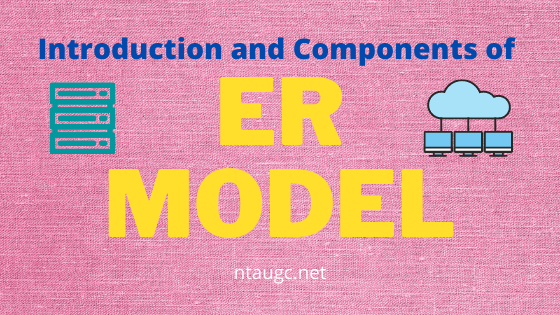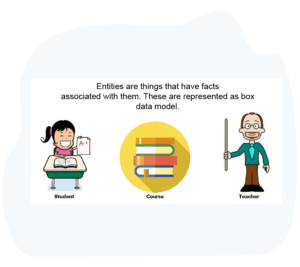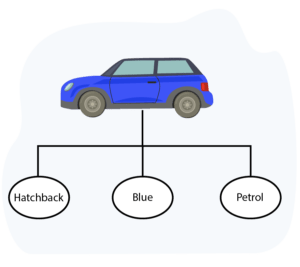# Introduction and Components of ER Model

• RDBMS## What is an ER Model Diagram?

• Entity-Relationship Model is a detailed conceptual diagram of the database that we are planning to create.
• It depicts a collection of real-world objects and the relationships between them.Let us consider ‘Teacher’ as one object and ‘Student’ as another.

The relationship between these two objects will be ‘to teach’.

Here, each ‘Teacher’ and ‘Student’ become the entities.‘Teaches’ is the relationship between them.

Each of the entities will have their own set of properties like name, age, contact number, etc.
We will refer to these properties as attributes of the entities.

## Why are ER diagrams helpful?

• The ER model was developed by Dr. Peter Chen in 1976 to simplify the task of understanding the data requirements.
• This, in turn, helps us to design a well-structured database.
With the help of ER diagrams, we can design our tables and the relationship between them.
• So we consider creating an ER Model as a best practice before implementing our database.
So we don’t need to acquire any extra technical knowledge to read ER diagrams.

## What does an ER model diagram comprise of?

ER model mainly comprises entities set, relations, and attributes.

• Entities: Real-world things that we are going to record in our databases.
• Entity Set: A collection of similar types of entities.
• Relationship: A connection between two entity sets.
• Attributes: Properties that describe the entities.

## Understanding the Entity

Entity is a:

1. Conceptual model of a tables
2. Conceptual model of a database
3. A logical model of a database
4. A logical model of tables

Ans:~ 2.Conceptual model of a database

## Understanding the Entity Set

For instance, consider designing a database for a university.We will need tables which store information about:

• All students of the university.
• All teachers of that university.

Here, the student table becomes one entity set and each student under this table becomes an entity.

♦ The table storing teacher’s details will become another entity set.

♦ In ER diagrams entity sets are represented using rectangles.

♦ To represent an entity set in an ER diagram we draw a rectangle containing the name of the entity set.

## Understanding AttributesThe image above is showing the different properties of the car.

• If we say that the car is an entity set then all its properties are known as the attributes.
• In ER diagrams attributes are represented by ellipses.
• Each ellipse represents one attribute.

## Understanding Relationships• Relationships show connections between two entity sets.
• The above image shows two entities: the car and the person.
• The diamond denotes the relationship between them.
• Here, it says that the car is owned by the person.
• Relationships are represented by a diamond and the name of the relationship is written inside the diamond.
• And the entities are connected to diamond by a line.

## KEY TAKEAWAYS

• An entity is a conceptual model of a database.
• The ER model is an object & real-world things
• An entity set is a collection of similar entities
• Age is a derived attribute as it can be calculated from the date of birth.
• Multivalued attributes are represented by double ellipses